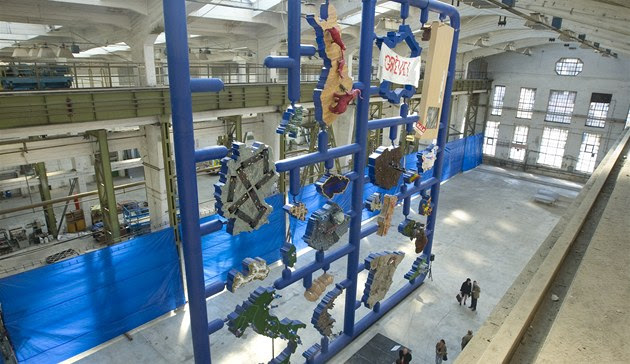## Thursday, August 11, 2011 ...//### BaBar: evidence for a charged Higgs bosonDuring the recent EPS-HEP 2011 conference in Grenoble, almost all particle physics viewers focused on the energy frontier i.e. the "Higgs and New Physics" category of the talks.

But it could have been a mistake for us to completely ignore other groups. For example "Flavor and Symmetries". A few hours ago, the Symmetry Magazine published an intriguing article by Lori Ann White:

Will the real Higgs boson please stand up?
This text about slim shady has led me to find the following talk by Manuel Franco Sevilla of SLAC's BaBar:
Semileptonic B and Charm Decays with BABAR (PDF)
And it was an interesting talk and paper, indeed.

Their goal was to measure an element of the CKM matrix,
$|V_{ub}|,$ which produces the mixing between the up quark and the lower partner of the charm quark. The Standard Model is fine. Recall that the complex phase in the CKM matrix is the only source of the CP-violation in the Standard Model which is why the elephant's flag on the bike also promotes CP-violation (scratched CP). This is the kind of physics that BaBar is relatively good at.

However, when you skip about 6 slides, you get to the measurement of the decays of some mesons:
$B\to D \tau \nu_\tau, \qquad B\to D^* \tau \nu_\tau$ The results are preliminary - when it comes to the procedures needed to approve the work. However, they already look intriguing (or they look intriguing so far).

The decaying $$B$$ meson is composed of a bottom quark $$b$$ and a light antiquark $$\bar q$$. It decays to $$D=c\bar q$$ - which carries a different charge than the $$B$$ - as well as a $$\tau^- \bar\nu_\tau$$ pair which carries the negative charge. Or the decay products may contain the antiparticles.

If you draw Feynman diagrams, the main tree level contributions just keep $$\bar q$$ unchanged. On the other hand, the $$b$$ quark emits either a $$W^-$$ - the charged gauge boson - or a $$H^-$$ - a charged Higgs boson. Any of these bosons may decay to the tau-neutrino pair while the original $$b$$ quark (bottom) becomes a $$c$$ quark (charm).

They decided to measure a ratio of two branching ratios (the numerator contains a $$\tau$$ while the denominator may contain any charged lepton instead) because various uncertainties cancel. The ratio is predicted by the Standard Model - which has no charged Higgs bosons - to be $$0.31\pm 0.02$$ or so. For the antiparticles, $$D^*$$, it is $$0.25\pm 0.02$$.

So the BaBar elephants are capable of finding the contribution of the virtual W-bosons to the ratio - all these things are statistically significant at much more than 5 sigma: they're able to see virtual W-boson-like particles.

However, they still see a 1.8 sigma excess in the ratio $$R$$ of the branching ratios above the Standard Model prediction that only allows a W-boson as the intermediate state. How does it translate to the knowledge about new physics? Take the 2010 Tanaka-Watanabe paper that calculates the theoretical predictions from MSSM. Look at Figure 1 on page 11.

The ratio $$R$$ is depicted as a function of $$x=\tan\beta / m_{H^\pm}$$ express in units $${\rm GeV}^{-1}$$ which are considered to be parameters of the Minimal Supersymmetric Standard Model - the most justified low-energy effective theory that actually contains some charged Higgs bosons.

Not surprisingly, for $$x=0$$, we reproduce $$R=0.32\pm 0.02$$ of the Standard Model. However, $$R$$ starts to decrease - for $$x=0.3$$, it is as small as $$R=0.15$$. If you want to return back to values of $$R$$ that are actually higher than $$0.32$$, like the observed $$R=0.456\pm 0.077$$, you need $$x\geq 0.45$$ or so.

So if you decide to believe the 1.8 sigma excess, which is of course a big If, this measurement is telling you that "almost certainly", the ratio
$\frac{\tan\beta}{m_{H^\pm}}$ is greater than 0.45 or so. This means that $$\tan\beta$$ is huge.

For example, if the mass of the charged Higgs boson were 100 GeV or so, $$\tan\beta$$ would have to exceed 40 or so - which already seems too high and excluded by the LHC at this moment. If the charged Higgs mass were substantially higher than 100 GeV, e.g. 200 GeV, you would need even higher $$\tan\beta$$ which seems crazy.

On the other hand, if you managed to have a charged Higgs boson at 50 GeV, which is not as impossible as you might think, the required $$\tan\beta$$ could be around 20 which is marginally plausible at this moment - as far as various LHC constraints go.

Of course, one shouldn't get carried away. It's absolutely plausible that the 1.8-sigma excess is just a fluke or the artifact of some mistakes in the theoretical or experimental work. But if you took the prior probabilities for the existence and absence of a charged Higgs boson to be equal and if you incorporated all evidence including 2-sigma "hints" in your calculations of the posterior probabilities, you would not find out that it is very likely that a charged Higgs boson actually exists but you would also see that its coupling has to be strictly separated from zero and/or its mass can't be too high.

At this vague level, the preliminary BaBar observation provides us with some circumstantial evidence supporting supersymmetry, too.

Off-topic: Entropa attracts tourists in Pilsen

As iDNES reported, a symbol of the Czech EU presidency, Entropa, has become a top sightseeing of the Techmania Science Museum here in Pilsen. I've seen it, too. It's huge.It has obtained a panel allowing the kids and other visitors to turn on/off various EU countries on the sculpture. Sometimes, some of the countries need to be repaired. Most recently, the Italian soccer players stopped masturbating so Italy had to be fixed.

Aside from the Italian do-it-yourself soccer stadium, Bulgaria as a Turkish toilet and Germany as a swastika-like superhighway are also among the popular countries. ;-)